New Features in Maple 2018 - Group Theory- Maplesoft# Group Theory

The Group Theory package includes an extensive collection of routines for constructing, computing with, and visualizing finitely generated groups, including permutation groups, finitely presented groups, Cayley table groups, “black-box” user defined groups, and abstract groups depending on symbolic parameters. Several new commands have been added to the Group Theory package in Maple 2018 for performing new tests and calculating more properties.

### New Subgroup Tests

• You can test whether a subgroup of a finite group is subnormal or permutable (quasi-normal) by using the new IsSubnormal and IsPermutable commands. These are generalizations of normal subgroups.
 > with( GroupTheory ):
 > G := Symm( 4 );> H := Subgroup( { Perm( [[1,2],[3,4]] ) }, G );> IsSubnormal( H, G );> IsPermutable( H, G );> IsNormal( H, G );> G := Symm( 3 );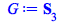> N := Subgroup( { Perm( [[1,2,3]] ) }, G );> IsNormal( N, G );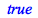> IsPermutable( N, G );> IsSubnormal( N, G );### Groups of Prime Power Order

• To check whether a finite group is a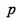-group, for a prime number, use the new IsPGroup command. The PGroupPrime command can be used to return the prime numberfor which a group is a finite-group.
 > IsPGroup( Symm( 3 ) );> IsPGroup( DihedralGroup( 4 ) );> PGroupPrime( DihedralGroup( 4 ) );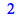> IsPGroup( DirectProduct( CyclicGroup( 128 ), QuaternionGroup() ) );### Other New and Updated Commands

• The ElementOrder command has been extended to work on elements of finite finitely presented groups.
 > G := < a, b | a^2, b^3, (a.b)^5 = 1 >:
 > ElementOrder( a.b^2 .a, G );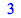• The new ElementPower command computes powers of elements of a permutation group or a Cayley table group.
• The new ClassNumber command returns the number of conjugacy classes of a finite group. In some cases, this can be faster than actually computing the conjugacy classes themselves and counting them.
 > ClassNumber( Symm( 3 ) );> ClassNumber( DirectProduct( Monster(), DihedralGroup( 4 * n ) ) ) assuming n :: posint;• The number of Abelian groups of a given order can be computed by using the new NumAbelianGroups command.
 > NumAbelianGroups( 1000 );## Ready for the next step?

*Free trials are not available for personal edition users.